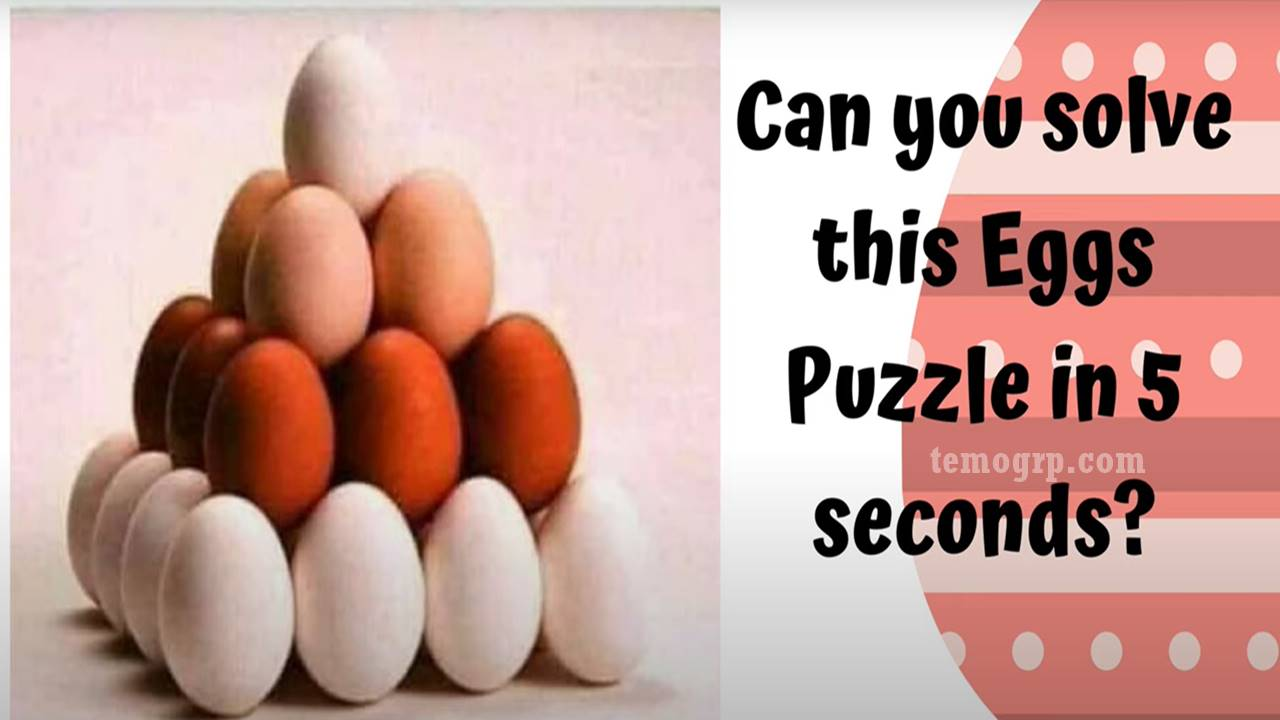Answer to the 'How Many Eggs Riddle Picture' on Facebook Brain Test or Quiz. How many eggs pyramid picture.answer. How many eggs 95% will fail answer?

Home >> >> >> Answer to How Many Eggs Riddle Picture Facebook Quiz/Brain Test

Many people are searching for the answer to the how many eggs riddle picture. This comes as no surprise as  95% will fail this brain test based on a pyramid picture or quiz..

How Many Eggs Puzzle is very confusing. That is why this viral riddle trends on Facebook, Instagram, TikTok, etc.

So, this article provides a definitive answer to understanding this puzzle.

### How Many Eggs are in the Picture Puzzle?

There are thirty (30) eggs in the picture riddle or puzzle. That is the correct answer.

## Answer to the 'How Many Eggs Riddle Picture' on Facebook Brain Test or Quiz

Here is the solution to how many eggs are in the picture puzzle:

• From Top Level: There is 1 egg.
• In The Second Level: There are 2×2 = 4 eggs.
• From The Third Level: There are 3×3 = 9 eggs.
• In The Base Level: There are 4×4 = 16 eggs.
• Final answer: 1+4+9+16= 30.

So, The correct answer to this tricky puzzle is 30 Eggs.

## Conclusion

This Maths brain test is one of those quizzes trending on social media today. Many folks share different views and opinions. Have you seen the puzzle before? Comment below.Name

ltr
item
Temo Group: Answer to How Many Eggs Riddle Picture Facebook Quiz/Brain Test
Answer to How Many Eggs Riddle Picture Facebook Quiz/Brain Test
Answer to the 'How Many Eggs Riddle Picture' on Facebook Brain Test or Quiz. How many eggs pyramid picture.answer. How many eggs 95% will fail answer?